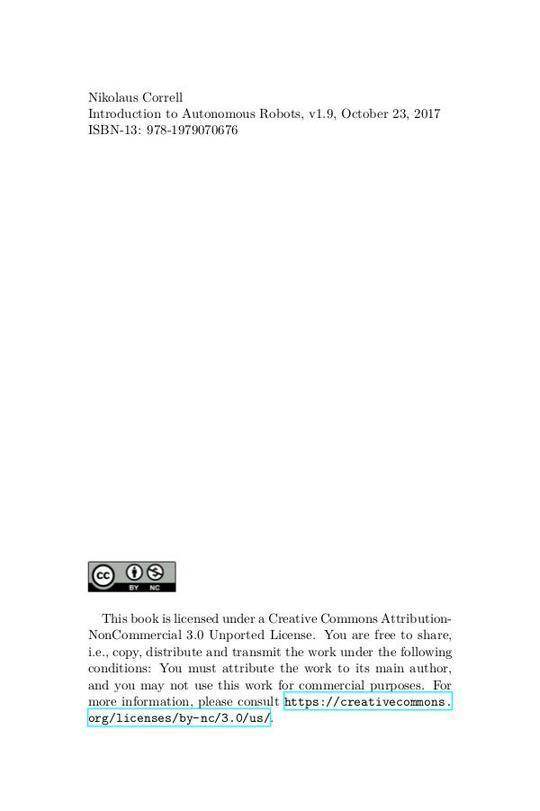Free

# book_CN

By Unknown
Free
Book Description
• 引言
• 智能与实例
• 一个机器人研究者的问题
• Ratslife
• 移动自主机器人的挑战
• 自主操作的挑战
• 运动和操作
• 运动和操作实例
• 静态和动态稳定性
• 自由度
• 正运动学和逆运动学
• 坐标系和参考系
• 矩阵符号
• 从一个坐标系映射到另一个坐标系
• 变换算术
• 其他的方向表示
• 所选机制的正运动学
• 一个简单的手臂的正运动学
• 差速轮机器人的正运动学
• 类似汽车转向的正运动学
• 使用Denavit-Hartenberg方案的正运动学
• 一些机制的反运动学
• 可解性
• 简单操作臂的逆运动学
• 移动机器人逆运动学
• 使用反馈控制的逆运动学
• 移动机器人的反馈控制
• 逆雅可比技术
• 路径规划
• 地图表示
• 路径规划算法
• 机器人实例
• Dijkstra算法
• A*
• 基于采样的路径规划
• 基本算法
• 将顶点与树相连
• 碰撞检测
• 路径平滑
• 在规模不同的尺度上规划
• 其他路径规划应用
• 总结和展望
• 传感器
• 机器人传感器
• 机器人运动学和内力的本体感知
• 基于光的传感器
• 反射
• 相移
• 飞行时间
• 基于声音的传感器
• 超声距离传感器
• 纹理识别
• 基于惯量的传感器
• 加速度计
• 陀螺仪
• 基于信标的传感器
• 术语
• 视觉
• 作为二维信号的图像
• 从信号到信息
• 基本的图像操作
• 基于卷积的滤波器
• 基于阈值的操作
• 形态操作
• 特征提取
• 把特征检测看作信息减少问题
• 特征
• 直线识别
• 使用最小二乘法的直线拟合
• 分割和合并算法
• 随机抽样与共识（Random Sample and Consensus，RANSAC）
• 霍夫变换
• 尺度不变特征变换
• 概述
• 使用比例不变特征的对象识别
• 不确定性和误差传播
• 机器人中的不确定性作为随机变量
• 误差传播
• 示例：直线拟合
• 示例：里程计
• 课后补充
• 定位
• 动机示例
• 马尔可夫定位
• 感知更新
• 动作更新
• 小结和示例
• 粒子滤波器
• 卡尔曼滤波器
• 基于概率地图的定位
• 最优的传感器融合
• 对预测与更新积分：卡尔曼滤波器
• 扩展卡尔曼滤波器
• 使用卡尔曼滤波器的方程式
• 抓取
• 如何找到成功的抓取？
• 同步定位与建图（SLAM）
• 引言
• 特殊情况一：单一特征
• 特殊情况二：两个特征
• 协方差矩阵
• EKF SLAM
• 基于图的SLAM
• SLAM作为最大似然估计问题
• 基于图SLAM的数值技术
• RGB-D SLAM
• 将距离数据转换为点云数据
• 迭代最近点（ICP）算法
• 匹配点
• 点对的权重
• 抛弃点对
• 误差度量和最小化算法
• RGB-D建图
• 三角法
• 逆三角法
• 三角等式
• 线性代数
• 点积
• 叉积
• 矩阵乘法
• 矩阵的逆
• 统计
• 随机变量和概率分布
• 正态分布
• 二维正态分布
• 条件概率和贝叶斯规则
• 两个随机过程的和
• 独立高斯随机变量的线性组合
• 检验统计意义
• 分布上的空假设
• 测试两个分布是否独立
• 真假测试的统计显著性
• 小结
• 如何写研究论文
• 原创研究
• 假设：或者，我们从这项工作中学到什么？
• 综述和教程
• 写出来！
• 样本课程
• 自主移动机器人简介
• 概述
• 材料
• 内容
• 自主操作介绍
• 概述
• 材料
• 内容
• 课堂辩论
• 参考文献
• 索引
No review for this book yet, be the first to review.
No comment for this book yet, be the first to comment
You May Also Like